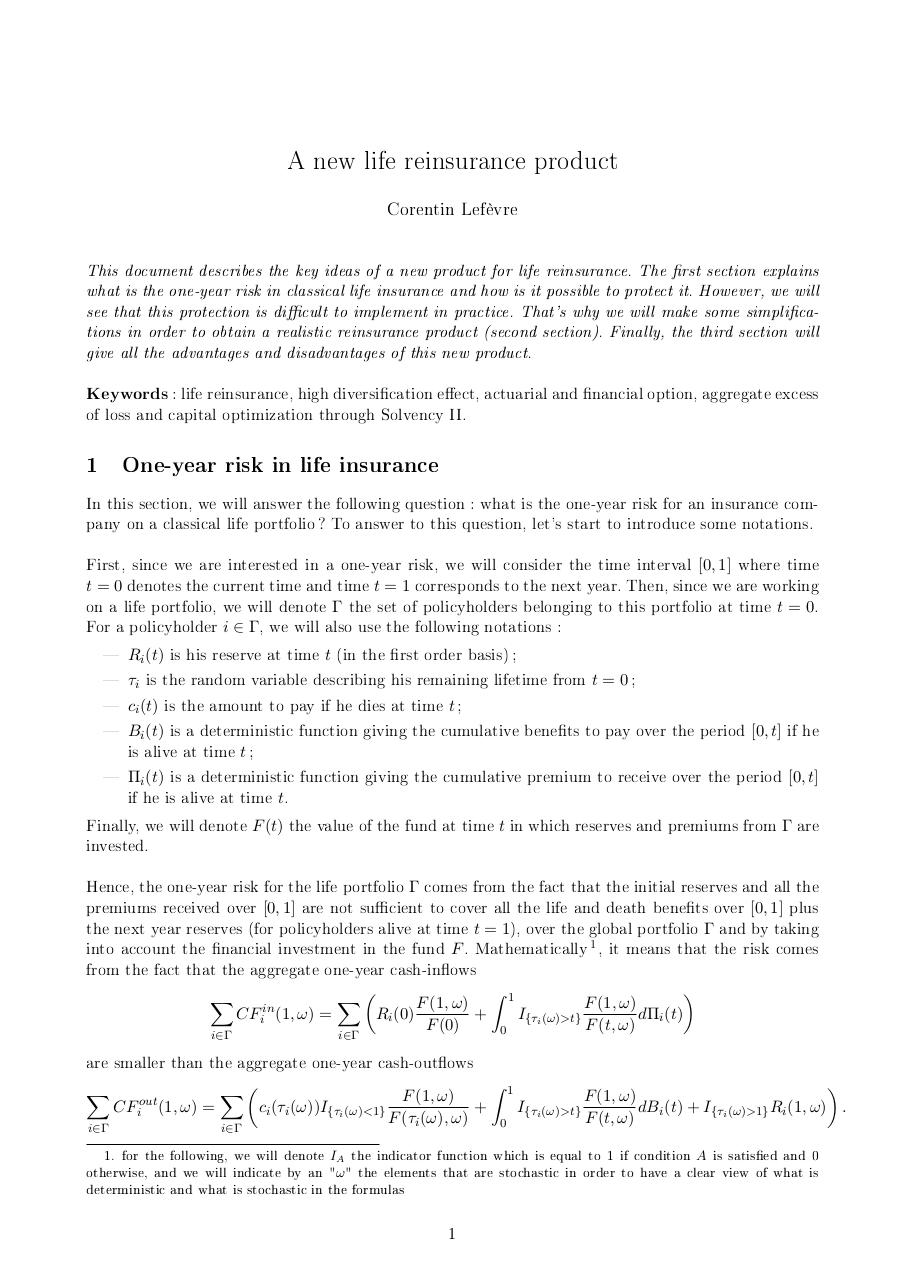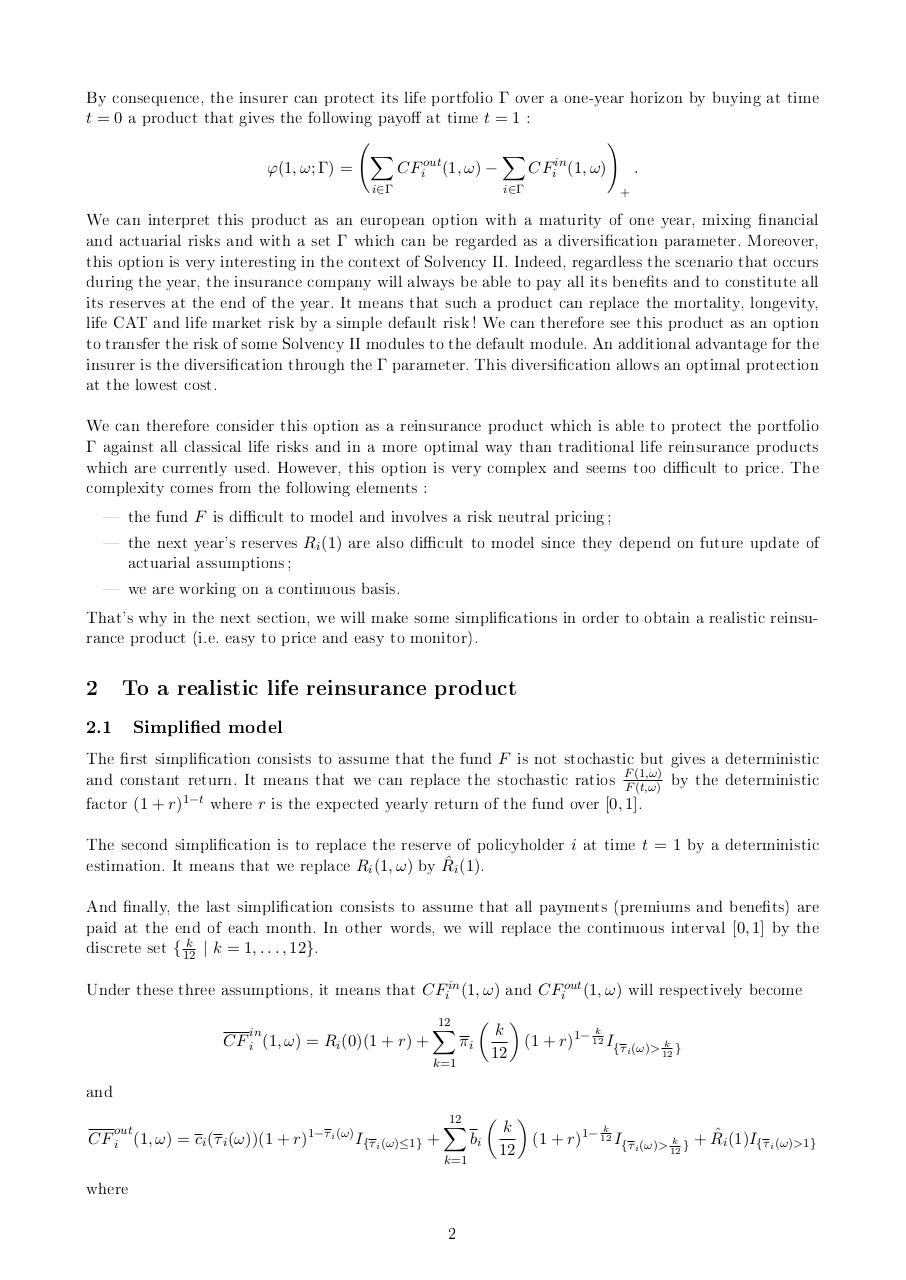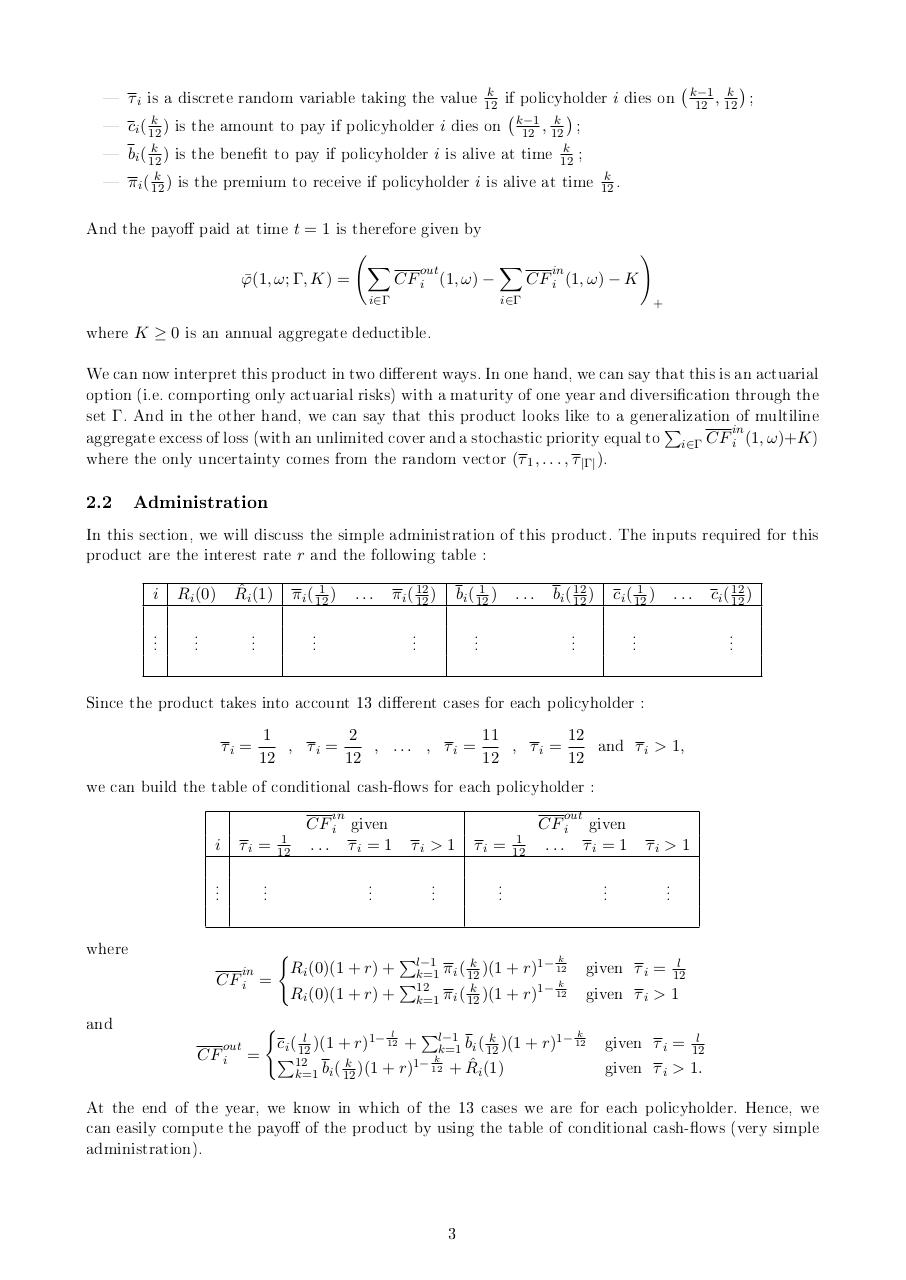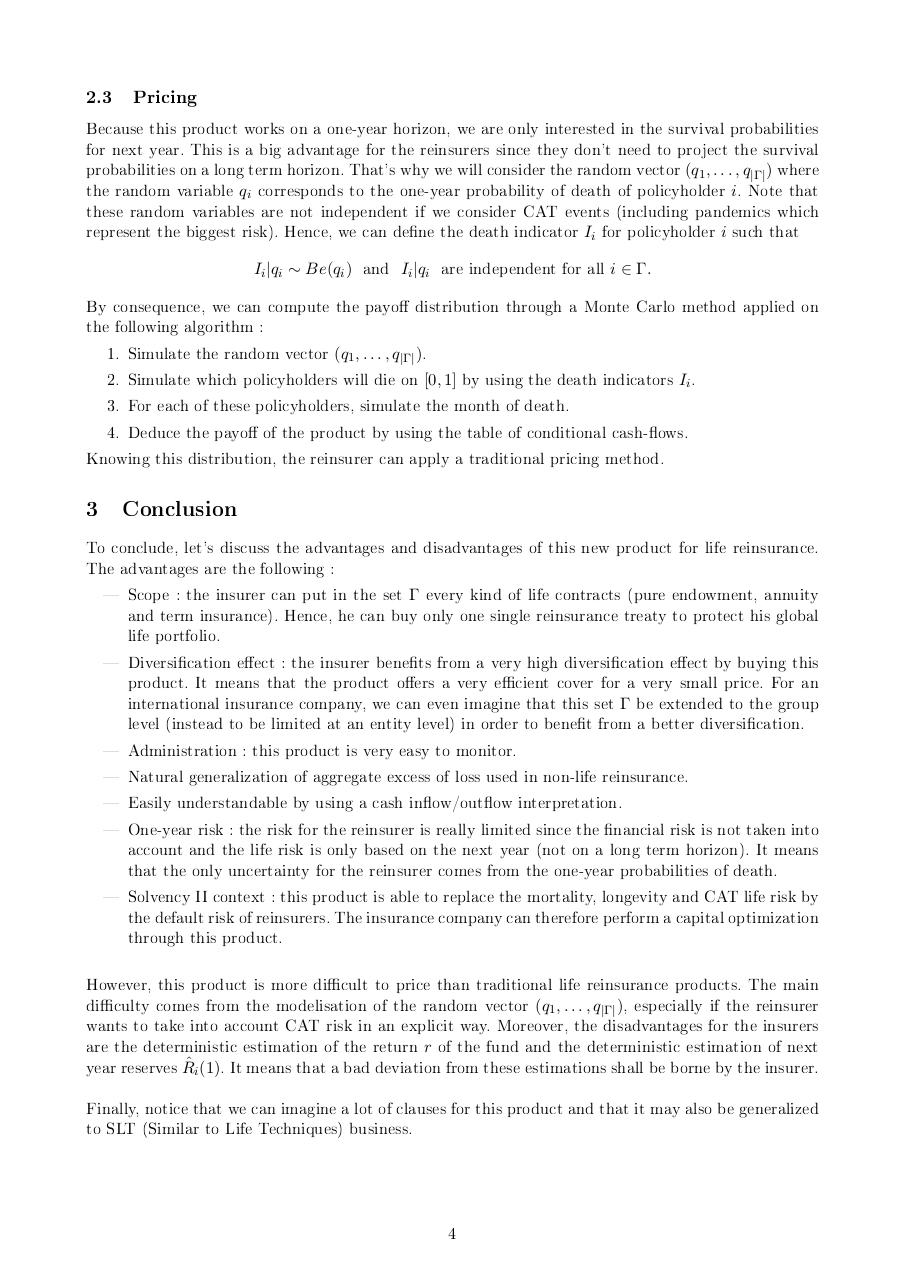# life reinsurance .pdf

### File information

Original filename: life_reinsurance.pdf

This PDF 1.5 document has been generated by TeX / MiKTeX pdfTeX-1.40.14, and has been sent on pdf-archive.com on 05/08/2017 at 14:29, from IP address 213.213.x.x. The current document download page has been viewed 598 times.
File size: 182 KB (4 pages).
Privacy: public file

life_reinsurance.pdf (PDF, 182 KB)

### Document preview

A new life reinsurance product
Corentin Lefèvre
This document describes the key ideas of a new product for life reinsurance. The rst section explains
what is the one-year risk in classical life insurance and how is it possible to protect it. However, we will
see that this protection is dicult to implement in practice. That's why we will make some simplications in order to obtain a realistic reinsurance product (second section). Finally, the third section will

Keywords : life reinsurance, high diversication eect, actuarial and nancial option, aggregate excess

of loss and capital optimization through Solvency II.
1

One-year risk in life insurance

In this section, we will answer the following question : what is the one-year risk for an insurance company on a classical life portfolio? To answer to this question, let's start to introduce some notations.
First, since we are interested in a one-year risk, we will consider the time interval [0, 1] where time
t = 0 denotes the current time and time t = 1 corresponds to the next year. Then, since we are working
on a life portfolio, we will denote Γ the set of policyholders belonging to this portfolio at time t = 0.
For a policyholder i ∈ Γ, we will also use the following notations :
 R (t) is his reserve at time t (in the rst order basis);
 τ is the random variable describing his remaining lifetime from t = 0 ;
 c (t) is the amount to pay if he dies at time t ;
 B (t) is a deterministic function giving the cumulative benets to pay over the period [0, t] if he
is alive at time t ;
 Π (t) is a deterministic function giving the cumulative premium to receive over the period [0, t]
if he is alive at time t.
Finally, we will denote F (t) the value of the fund at time t in which reserves and premiums from Γ are
invested.
Hence, the one-year risk for the life portfolio Γ comes from the fact that the initial reserves and all the
premiums received over [0, 1] are not sucient to cover all the life and death benets over [0, 1] plus
the next year reserves (for policyholders alive at time t = 1), over the global portfolio Γ and by taking
into account the nancial investment in the fund F . Mathematically , it means that the risk comes
from the fact that the aggregate one-year cash-inows
i

i

i

i

i

1

X

CFiin (1, ω)

i∈Γ


Z 1
X
F (1, ω)
F (1, ω)
=
Ri (0)
+
I{τi (ω)&gt;t}
dΠi (t)
F (0)
F (t, ω)
0
i∈Γ

are smaller than the aggregate one-year cash-outows
X
i∈Γ

CFiout (1, ω) =


Z 1
X
F (1, ω)
F (1, ω)
ci (τi (ω))I{τi (ω)&lt;1}
+
I{τi (ω)&gt;t}
dBi (t) + I{τi (ω)&gt;1} Ri (1, ω) .
F (τi (ω), ω)
F (t, ω)
0
i∈Γ

1. for the following, we will denote IA the indicator function which is equal to 1 if condition A is satised and 0
otherwise, and we will indicate by an "ω " the elements that are stochastic in order to have a clear view of what is
deterministic and what is stochastic in the formulas

1

By consequence, the insurer can protect its life portfolio Γ over a one-year horizon by buying at time
t = 0 a product that gives the following payo at time t = 1 :
!
ϕ(1, ω; Γ) =

X

CFiout (1, ω) −

i∈Γ

X

CFiin (1, ω)

i∈Γ

.
+

We can interpret this product as an european option with a maturity of one year, mixing nancial
and actuarial risks and with a set Γ which can be regarded as a diversication parameter. Moreover,
this option is very interesting in the context of Solvency II. Indeed, regardless the scenario that occurs
during the year, the insurance company will always be able to pay all its benets and to constitute all
its reserves at the end of the year. It means that such a product can replace the mortality, longevity,
life CAT and life market risk by a simple default risk! We can therefore see this product as an option
to transfer the risk of some Solvency II modules to the default module. An additional advantage for the
insurer is the diversication through the Γ parameter. This diversication allows an optimal protection
at the lowest cost.
We can therefore consider this option as a reinsurance product which is able to protect the portfolio
Γ against all classical life risks and in a more optimal way than traditional life reinsurance products
which are currently used. However, this option is very complex and seems too dicult to price. The
complexity comes from the following elements :
 the fund F is dicult to model and involves a risk neutral pricing;
 the next year's reserves R (1) are also dicult to model since they depend on future update of
actuarial assumptions;
 we are working on a continuous basis.
That's why in the next section, we will make some simplications in order to obtain a realistic reinsurance product (i.e. easy to price and easy to monitor).
i

2

To a realistic life reinsurance product

2.1 Simplied model

The rst simplication consists to assume that the fund F is not stochastic but gives a deterministic
and constant return. It means that we can replace the stochastic ratios
by the deterministic
factor (1 + r) where r is the expected yearly return of the fund over [0, 1].
The second simplication is to replace the reserveˆ of policyholder i at time t = 1 by a deterministic
estimation. It means that we replace R (1, ω) by R (1).
And nally, the last simplication consists to assume that all payments (premiums and benets) are
paid at the end of each month. In other words, we will replace the continuous interval [0, 1] by the
discrete set { | k = 1, . . . , 12}.
Under these three assumptions, it means that CF (1, ω) and CF (1, ω) will respectively become
F (1,ω)
F (t,ω)

1−t

i

i

k
12

in
i

in
CF i (1, ω)

= Ri (0)(1 + r) +

where


πi

k=1

and
out
CF i (1, ω)

12
X

out
i

= ci (τ i (ω))(1 + r)

1−τ i (ω)

I{τ i (ω)≤1} +

12
X
k=1

2

k
12


bi



k
12

k

(1 + r)1− 12 I{τ i (ω)&gt; k }
12



k
ˆ i (1)I{τ (ω)&gt;1}
(1 + r)1− 12 I{τ i (ω)&gt; k } + R
i
12

 τ is a discrete random variable taking the value if policyholder
i dies on

 c ( ) is the amount to pay if policyholder i dies on , ;
 b ( ) is the benet to pay if policyholder i is alive at time ;
 π ( ) is the premium to receive if policyholder i is alive at time .
And the payo paid at time t = 1 is therefore given by
k
12

i

k−1 k
12 , 12



;

k−1 k
12 12
k
12

k
i 12
k
i 12
k
i 12

k
12

!
ϕ(1,
¯ ω; Γ, K) =

out
CF i (1, ω)

X

X

in
CF i (1, ω)

−K

i∈Γ

i∈Γ

+

where K ≥ 0 is an annual aggregate deductible.
We can now interpret this product in two dierent ways. In one hand, we can say that this is an actuarial
option (i.e. comporting only actuarial risks) with a maturity of one year and diversication through the
set Γ. And in the other hand, we can say that this product looks like to a generalization
of multiline
aggregate excess of loss (with an unlimited cover and a stochastic priority equal to P CF (1, ω)+K )
where the only uncertainty comes from the random vector (τ , . . . , τ ).
i∈Γ

1

in
i

|Γ|

In this section, we will discuss the simple administration of this product. The inputs required for this
product are the interest rate r and the following table :
i

Ri (0)

ˆ i (1)
R

..

..

..

1
π i ( 12
)

...

..

π i ( 12
12 )

1
bi ( 12
)

..

bi ( 12
12 )

...

..

1
ci ( 12
)

..

...

..

ci ( 12
12 )

..

Since the product takes into account 13 dierent cases for each policyholder :
1
2
11
12
τ =
, τ =
, ... , τ =
, τ =
and τ &gt; 1,
12
12
12
12
we can build the table of conditional cash-ows for each policyholder :
CF given
CF
given
i

i

i

τi =

..

..

where
in
CF i

and

1
12

i

i

in
i

...

τi = 1

τi &gt; 1

..

..

τi =

..

1
12

i

out
i

...

τi = 1

τi &gt; 1

..

..

(
P
k
1− 12
k
Ri (0)(1 + r) + l−1
k=1 π i ( 12 )(1 + r)
=
P
k
1− 12
k
Ri (0)(1 + r) + 12
k=1 π i ( 12 )(1 + r)

given
given

τi =

l
12

τi &gt; 1

given τ =
given τ &gt; 1.
At the end of the year, we know in which of the 13 cases we are for each policyholder. Hence, we
can easily compute the payo of the product by using the table of conditional cash-ows (very simple
out
CF i

(
P
l
k
1− 12
l
k
ci ( 12
)(1 + r)1− 12 + l−1
k=1 bi ( 12 )(1 + r)
= P12
k
1− 12
k
ˆ i (1)
+R
k=1 bi ( 12 )(1 + r)

3

i
i

l
12

2.3 Pricing

Because this product works on a one-year horizon, we are only interested in the survival probabilities
for next year. This is a big advantage for the reinsurers since they don't need to project the survival
probabilities on a long term horizon. That's why we will consider the random vector (q , . . . , q ) where
the random variable q corresponds to the one-year probability of death of policyholder i. Note that
these random variables are not independent if we consider CAT events (including pandemics which
represent the biggest risk). Hence, we can dene the death indicator I for policyholder i such that
I |q ∼ Be(q ) and I |q are independent for all i ∈ Γ.
By consequence, we can compute the payo distribution through a Monte Carlo method applied on
the following algorithm :
1. Simulate the random vector (q , . . . , q ).
2. Simulate which policyholders will die on [0, 1] by using the death indicators I .
3. For each of these policyholders, simulate the month of death.
4. Deduce the payo of the product by using the table of conditional cash-ows.
Knowing this distribution, the reinsurer can apply a traditional pricing method.
1

|Γ|

i

i

i

i

i

i

1

i

|Γ|

i

3

Conclusion

To conclude, let's discuss the advantages and disadvantages of this new product for life reinsurance.
The advantages are the following :
 Scope : the insurer can put in the set Γ every kind of life contracts (pure endowment, annuity
and term insurance). Hence, he can buy only one single reinsurance treaty to protect his global
life portfolio.
 Diversication eect : the insurer benets from a very high diversication eect by buying this
product. It means that the product oers a very ecient cover for a very small price. For an
international insurance company, we can even imagine that this set Γ be extended to the group
level (instead to be limited at an entity level) in order to benet from a better diversication.
 Administration : this product is very easy to monitor.
 Natural generalization of aggregate excess of loss used in non-life reinsurance.
 Easily understandable by using a cash inow/outow interpretation.
 One-year risk : the risk for the reinsurer is really limited since the nancial risk is not taken into
account and the life risk is only based on the next year (not on a long term horizon). It means
that the only uncertainty for the reinsurer comes from the one-year probabilities of death.
 Solvency II context : this product is able to replace the mortality, longevity and CAT life risk by
the default risk of reinsurers. The insurance company can therefore perform a capital optimization
through this product.
However, this product is more dicult to price than traditional life reinsurance products. The main
diculty comes from the modelisation of the random vector (q , . . . , q ), especially if the reinsurer
wants to take into account CAT risk in an explicit way. Moreover, the disadvantages for the insurers
are the deterministic
estimation of the return r of the fund and the deterministic estimation of next
ˆ
year reserves R (1). It means that a bad deviation from these estimations shall be borne by the insurer.
Finally, notice that we can imagine a lot of clauses for this product and that it may also be generalized
to SLT (Similar to Life Techniques) business.
1

i

4

|Γ|#### HTML Code

Copy the following HTML code to share your document on a Website or Blog

#### QR Code### Related keywords